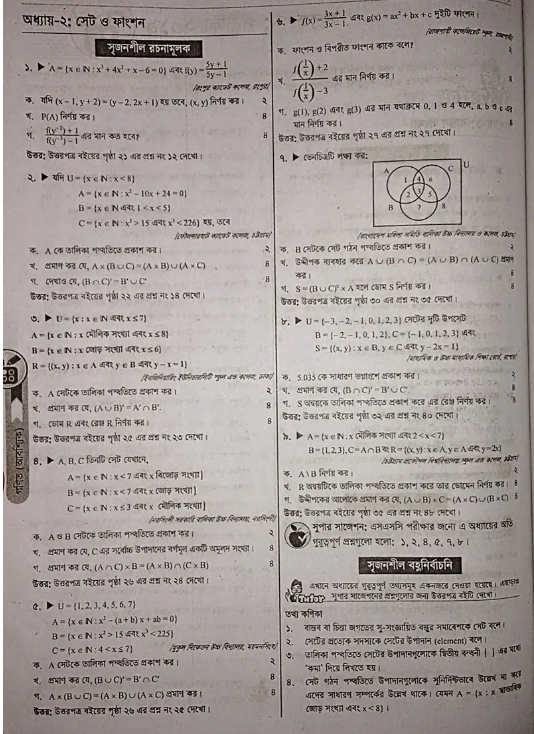# SSC General Math suggestion 2023 in Bangladesh

Are you looking for the SSC General Math suggestion 2023 in Bangladesh?The Secondary School Certificate, along with known as SSC, is a public examination taken by students in Bangladesh after successful achievement of ten years of schooling.

There are two types of math in SSC. One is general mathematics and the other is higher mathematics. However general mathematics is compulsory for both science, commerce, and art students whereas higher mathematics is only for science students.

## Mark Distribution of SSC General Math 2023:

The pattern of the question must be by SSC Mathematics Syllabus 2023. According to the syllabus, the total mark is one hundred for this subject. The broad question of Bangladesh is changing all the time. There are two parts to the 2023 math in-board question. And one is written, and the other is MCQ. The marks were also very carefully given out. The first 70 marks for the Writing Department so all students can pass the exam.  Besides, number 30 is saved for the MCQ portion. If you want to get a GPA 5  in the exam, then you should do well in both segments. Here I am offering some suggestions for SSC candidates.

## The SSC General Math suggestion 2023 is given below:

Here we will give you the SSC General Math suggestion 2023  for you. If you fathom them very well, Confidently, you will be able to answer the complete questions and efficiently do well in the exam.

Chapter One: Real Numbers

Exercise 01: 20(b,c,e),22

Chapter Two: Sets and Functions

Exercise 2.1 : 1,4, 6, 9, 10, 11, (Example12,15)

Exercise 2.2 : 10, 12, 13, 15, 18, 23(Example 25)

Chapter  Three: Algebraic Equations

Exercise 3.1 : 8, 9, 10, 13,15, (Examples 7,8, 11)

Exercise 3.2 : 4, 7, 13, 15, 16, (Examples 16)

Exercise 3.3 : 5, 6, 18, 21, 25.(Examples 29)

Exercise 3.5 : 14, 17, 23, 32, (Example 32)

Chapter Four: Exponents and Logarithms

Exercise 4.1 : 4, 6, 8, 15, 16, 18, (Example 4)

Exercise 4.2 : 1(a), 2(b), 3(c), 4(a), 5,( Example 6,7(b),10)

Chapter Seven : Practical Geometry

Exercise 7.1 : 6.

Exercise 7.2: 4,15.

Edit 1, 2, 3, 5, 7, 9, 11 (Example 2, 3)

Chapter Eight: The Circle

Exercise 8.1 : 3, 4, 6, 8, 12.

Exercise 8.2 : 3, 4, 5.

Exercise 8.5 : 11, 13, 15, 16.

Theorem 15 (Example 1 on page 131), 17, 18, 22, 23.

Chapter Nine: Trigonometric Ratios

Exercise 9.1 : 5, 11, 17, 21, (examples 10, 11)

Exercise 9.2 : 20, 24, 26, 29, (Example 14)

Chapter Ten: Distance and Time

Exercise 10: 10,13,14,17.

Chapter Thirteen: The Finite Clause

Exercise 13.1 : 8, 15, 16, 21, 23 (Examples 3, 5)

Exercise 13.2 : 8, 9, 11, 15, 23.

Chapter Sixteen: Limitation

Exercise 16.1 : 5, 6.

Exercise 16.2 : 3, 5, 10, 13, (Example 16)

Exercise 16.3 : 2, 5, 6, (Example 27)

Exercise 16.4 : 11, 19, (Example 32)

Chapter Seventeen: Statistics

Exercise 17 : 10, 12, 14, 15 (Examples 4,7)Your assignment is to solve our important questions from the exercises mentioned above.

Every segment of the Math book is very supreme. But if one practices the chapters given here very well, then it is probable to achieve good marks in math in the upcoming exam. If you exercise the board book features well, then you will be able to answer any creative question in the exam. So, you should have to master the whole book very wisely.

In-sha-Allah, this will be common for you in the SSC 2023 exam.## SSC General Math suggestion Final Word

This absolute SSC General Math suggestion 2023 by our skillful teachers. You don’t need to run to another place to collect suggestions. Take preparation according to our suggestion. Thank you so much for reading this article. Good luck with your exam.

Thank you so much to those who have read out the whole article from the beginning. We publish all the information related to education on our website.